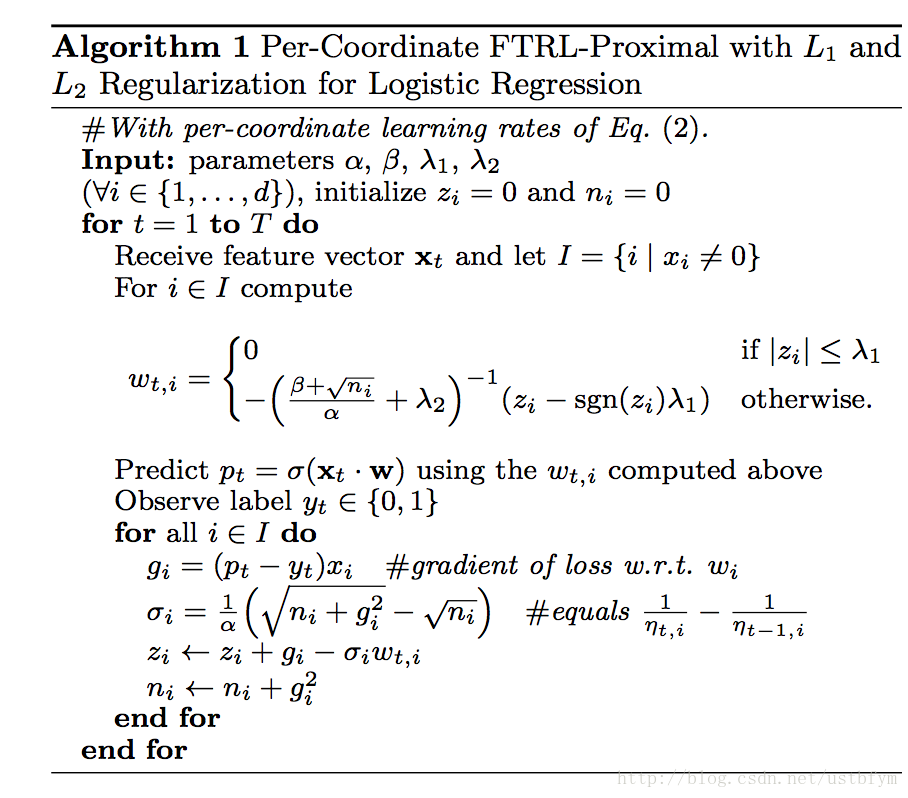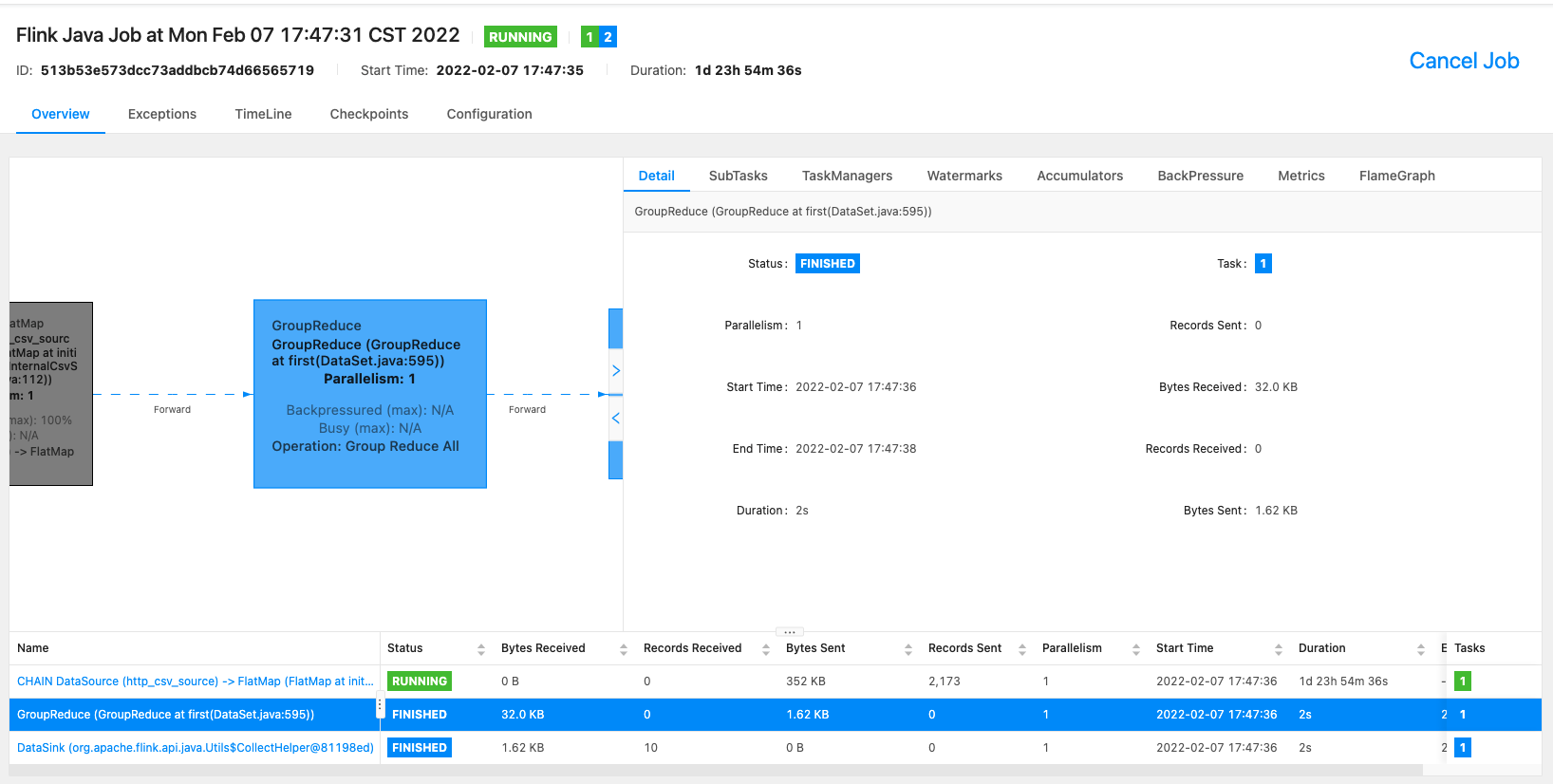# 在线学习 FTRL 介绍及基于 Flink 实现在线学习流程｜社区征文

• 2022 年 2 月 09 日
• 本文字数：5914 字

阅读完需：约 19 分钟# FTRL 及工程实现

## FTRL 介绍

FTR 是 FTRL 的前身，思想是每次找到让之前所有样本的损失函数之和最小的参数。FTRL，即 Follow The Regularized Leader，借鉴经典的 TG，OGD , L1-FOBOS, L1-RDA 在之前的几个工作上产生的，主要出发点就是为了提高稀疏度且满足精度要求。FTRL 在 FTL 的优化目标的基础上，加入了正则化，防止过拟合。FTRL 的损失函数一般也不容易求解，这种情况下，一般需要找一个代理的损失函数。代理损失函数需要满足以下条件：

1. 代理损失函数比较容易求解，最好是有解析解。

2. 代理损失函数求得的解，和原函数的解的差距越小越好

## 工程实现

逻辑回归下的 per-coordinate FTRL_Proximal 的伪代码如下：四个参数的设定结合 paper 里的指导意见以及反复实验测试，找一组适合自己问题的参数就可以了。上面所谓的 per-coordinate，其意思是 FTRL 是对 w 每一维分开训练更新的，每一维使用的是不同的学习速率，也是上面代码中 lamda2 之前的那一项。与 w 所有特征维度使用统一的学习速率相比，这种方法考虑了训练样本本身在不同特征上分布的不均匀性，如果包含 w 某一个维度特征的训练样本很少，每一个样本都很珍贵，那么该特征维度对应的训练速率可以独自保持比较大的值，每来一个包含该特征的样本，就可以在该样本的梯度上前进一大步，而不需要与其他特征维度的前进步调强行保持一致。

## 开源实现

### 参考 Python 代码实现

# coding=utf-8import numpy as npclass LR(object):    @staticmethod    def fn(w, x):        '''决策函数为sigmoid函数        '''        return 1.0 / (1.0 + np.exp(-w.dot(x)))    @staticmethod    def loss(y, y_hat):        '''交叉熵损失函数        '''        return np.sum(np.nan_to_num(-y * np.log(y_hat) - (1 - y) * np.log(1 - y_hat)))    @staticmethod    def grad(y, y_hat, x):        '''交叉熵损失函数对权重w的一阶导数        '''        return (y_hat - y) * xclass FTRL(object):    def __init__(self, dim, l1, l2, alpha, beta, decisionFunc=LR):        self.dim = dim        self.decisionFunc = decisionFunc        self.z = np.zeros(dim)        self.n = np.zeros(dim)        self.w = np.zeros(dim)        self.l1 = l1        self.l2 = l2        self.alpha = alpha        self.beta = beta    def predict(self, x):        return self.decisionFunc.fn(self.w, x)    def update(self, x, y):        self.w = np.array([0 if np.abs(self.z[i]) <= self.l1 else (np.sign(            self.z[i]) * self.l1 - self.z[i]) / (self.l2 + (self.beta + np.sqrt(self.n[i])) / self.alpha) for i in xrange(self.dim)])        y_hat = self.predict(x)        g = self.decisionFunc.grad(y, y_hat, x)        sigma = (np.sqrt(self.n + g * g) - np.sqrt(self.n)) / self.alpha        self.z += g - sigma * self.w        self.n += g * g        return self.decisionFunc.loss(y, y_hat)    def train(self, trainSet, verbos=False, max_itr=100000000, eta=0.01, epochs=100):        itr = 0        n = 0        while True:            for x, y in trainSet:                loss = self.update(x, y)                if verbos:                    print "itr=" + str(n) + "\tloss=" + str(loss)                if loss < eta:                    itr += 1                else:                    itr = 0                if itr >= epochs:  # 损失函数已连续epochs次迭代小于eta                    print "loss have less than", eta, " continuously for ", itr, "iterations"                    return                n += 1                if n >= max_itr:                    print "reach max iteration", max_itr                    returnclass TestData(object):    def __init__(self, file, d):        self.d = d        self.file = file    def __iter__(self):        with open(self.file, 'r') as f_in:            for line in f_in:                arr = line.strip().split()                if len(arr) >= (self.d + 1):                    yield (np.array([float(x) for x in arr[0:self.d]]), float(arr[self.d]))if __name__ == '__main__':    d = 4    testData = TestData("train.txt", d)    ftrl = FTRL(dim=d, l1=1.0, l2=1.0, alpha=0.1, beta=1.0)    ftrl.train(testData, verbos=False, max_itr=100000, eta=0.01, epochs=100)    w = ftrl.w    print w    correct = 0    wrong = 0    for x, y in testData:        y_hat = 1.0 if ftrl.predict(x) > 0.5 else 0.0        if y == y_hat:            correct += 1        else:            wrong += 1    print "correct ratio", 1.0 * correct / (correct + wrong)

### 具体代码实现逻辑如下：

● 建立特征处理管道，其包括 StandardScaler 和 FeatureHasher，进行标准化缩放和特征哈希，最后得到了特征向量。

Pipeline featurePipeline = new Pipeline().add(new StandardScaler().setSelectedCols(numericalColNames)).add(new FeatureHasher().setSelectedCols(selectedColNames).setCategoricalCols(categoryColNames).setOutputCol(vecColName).setNumFeatures(numHashFeatures));// fit feature pipeline model// 构建特征工程流水线PipelineModel featurePipelineModel = featurePipeline.fit(trainBatchData);

● 准备数据集这里构建 kafka 之类的流式数据，并进行实时切分得到原始训练数据和原始预测数据，

// 准备流式数据集CsvSourceStreamOp data = new CsvSourceStreamOp().setFilePath("http://alink-release.oss-cn-beijing.aliyuncs.com/data-files/avazu-ctr-train-8M.csv").setSchemaStr(schemaStr).setIgnoreFirstLine(true);// 这里可以采用kafaka数据源 KafkaSourceStreamOp soure = new KafkaSourceStreamOp()                .setBootstrapServers("localhost:9092")                .setTopic("train_data_topic")                .setStartupMode("EARLIEST")                .setGroupId("");// 对于流数据源进行实时切分得到原始训练数据和原始预测数据SplitStreamOp splitter = new SplitStreamOp().setFraction(0.5).linkFrom(data);

● 训练出一个逻辑回归模型作为 FTRL 算法的初始模型，这是为了系统冷启动的需要。

LogisticRegressionTrainBatchOp lr = new LogisticRegressionTrainBatchOp().setVectorCol(vecColName).setLabelCol(labelColName).setWithIntercept(true).setMaxIter(10);BatchOperator<?> initModel = featurePipelineModel.transform(trainBatchData).link(lr);

● 在初始模型基础上进行 FTRL 在线训练；

// 在初始模型基础上进行FTRL在线训练       FtrlTrainStreamOp model = new FtrlTrainStreamOp(initModel).setVectorCol(vecColName).setLabelCol(labelColName).setWithIntercept(true).setAlpha(0.1).setBeta(0.1).setL1(0.01).setL2(0.01).setTimeInterval(10).setVectorSize(numHashFeatures).linkFrom(featurePipelineModel.transform(splitter));

● 在 FTRL 在线模型的基础上，连接预测数据进行预测；/

FtrlPredictStreamOp predictResult = new FtrlPredictStreamOp(initModel)   .setVectorCol(vecColName)                .setPredictionCol("pred")  .setReservedCols(new String[]{labelColName})  .setPredictionDetailCol("details")  .linkFrom(model, featurePipelineModel.transform(splitter.getSideOutput(0)));

● 对预测结果流进行评估

// 对预测结果流进行评估predictResult.link( new EvalBinaryClassStreamOp().setLabelCol(labelColName).setPredictionCol("pred")   .setPredictionDetailCol("details").setTimeInterval(10)).link(new JsonValueStreamOp()   .setSelectedCol("Data")   .setReservedCols(new String[]{"Statistics"}).setOutputCols(new String[]{"Accuracy", "AUC", "ConfusionMatrix"}).setJsonPath(new String[]{".AUC", "\$.ConfusionMatrixx"})  )  .print();StreamOperator.execute();

### 编译打包

wget https://archive.apache.org/dist/flink/flink-1.13.0/flink-1.13.0-bin-scala_2.11.tgztar -xf flink-1.13.0-bin-scala_2.11.tgz && cd flink-1.13.0./bin/start-cluster.sh

./bin/flink run -p 1 -c org.example.FTRLExample  FlinkOnlineProject-1.0-SNAPSHOT.jar  

### 查看任务状态# 工程优化手段

## 内存优化

L1 范数加策略，训练结果 w 很稀疏，在用 w 做 predict 的时候节省了内存

1. 在线丢弃训练数据中很少出现的特征(probabilistic feature inclusion）

2. 浮点数重新编码

3. 训练若干相似的模型，保证可以部分共享相关特征。

4. 单值结构，多个模型公用一个特征存储，同时更新这个共有的特征结构

5. 使用正负样本的数量来计算梯度的和

6. 抽样训练集，选择更有价值的的样本

# 参考文档## 评论